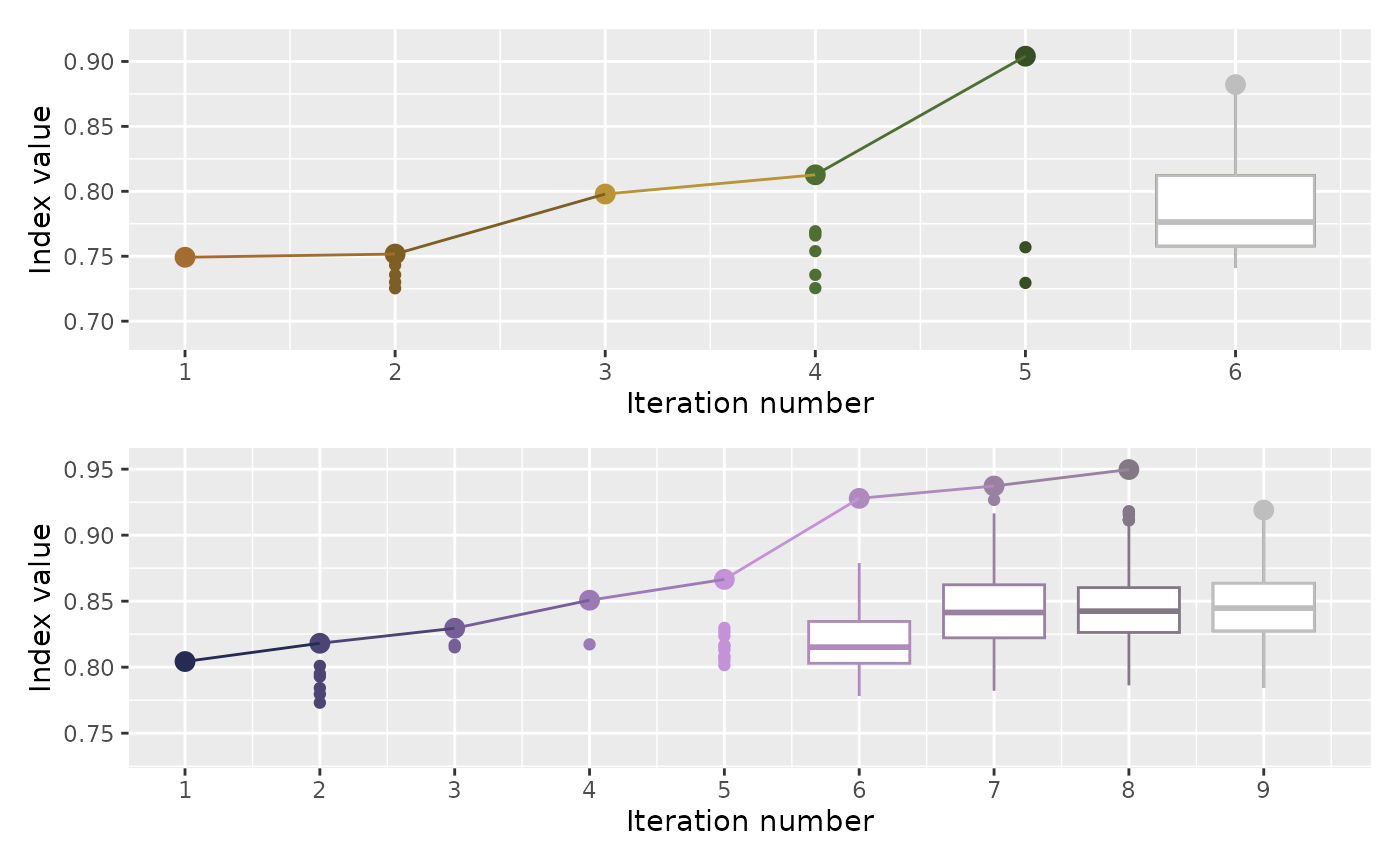Plot the count in each iteration

explore_trace_search(
dt,
iter = NULL,
color = NULL,
cutoff = 15,
extend_lower = 0.95,
...
)

## Arguments

dt

a data object collected by the projection pursuit guided tour optimisation in tourr

iter

the variable to be plotted on the x-axis

color

the variable to be coloured by

cutoff

numeric; if the number of searches in one iteration is smaller than cutoff, a point geom, rather than boxplot geom, will be used.

extend_lower

a numeric for extending the y-axis to display text labels

...

arguments passed into geom_label_repel() for displaying text labels

## Value

a ggplot object for diagnosing how many points the optimiser(s) have searched

Other main plot functions: explore_space_pca(), explore_space_tour(), explore_trace_interp()

## Examples

# Summary plots for search points in two algorithms
library(patchwork)
library(dplyr)
library(ggplot2)
p1 <- holes_1d_better %>% explore_trace_search() +
scale_color_continuous_botanical(palette = "fern")
#> map tries to the x-axis
#> map tries to color
p2 <- holes_2d_better_max_tries %>% explore_trace_search() +
scale_color_continuous_botanical(palette = "daisy")
#> map tries to the x-axis
#> map tries to color
p1 / p2